<![CDATA[Combinatorics, Probability and Computing]]>https://www.cambridge.org/core/journals/combinatorics-probability-and-computing/latest-issueCombinatorics, Probability and Computing0963548314692163Cambridge University Press<![CDATA[Distribution of tree parameters by martingale approach]]>

For a uniform random labelled tree, we find the limiting distribution of tree parameters which are stable (in some sense) with respect to local perturbations of the tree structure. The proof is based on the martingale central limit theorem and the Aldous–Broder algorithm. In particular, our general result implies the asymptotic normality of the number of occurrences of any given small pattern and the asymptotic log-normality of the number of automorphisms.

]]>
https://dx.doi.org/10.1017/S0963548321000523?rft_dat=source%3Ddrssdoi:10.1017/S0963548321000523Distribution of tree parameters by martingale approach73776410.1017/S0963548321000523https://dx.doi.org/10.1017/S0963548321000523?rft_dat=source%3Ddrss2022-04-29Combinatorics, Probability and Computing2022-04-29Isaev, MikhailSouthwell, AngusZhukovskii, Maksim
<![CDATA[Tuning as convex optimisation: a polynomial tuner for multi-parametric combinatorial samplers]]>

Combinatorial samplers are algorithmic schemes devised for the approximate- and exact-size generation of large random combinatorial structures, such as context-free words, various tree-like data structures, maps, tilings, RNA molecules. They can be adapted to combinatorial specifications with additional parameters, allowing for a more flexible control over the output profile of parametrised combinatorial patterns. One can control, for instance, the number of leaves, profile of node degrees in trees or the number of certain sub-patterns in generated strings. However, such a flexible control requires an additional and nontrivial tuning procedure. Using techniques of convex optimisation, we present an efficient tuning algorithm for multi-parametric combinatorial specifications. Our algorithm works in polynomial time in the system description length, the number of tuning parameters, the number of combinatorial classes in the specification, and the logarithm of the total target size. We demonstrate the effectiveness of our method on a series of practical examples, including rational, algebraic, and so-called Pólya specifications. We show how our method can be adapted to a broad range of less typical combinatorial constructions, including symmetric polynomials, labelled sets and cycles with cardinality lower bounds, simple increasing trees or substitutions. Finally, we discuss some practical aspects of our prototype tuner implementation and provide its benchmark results.

]]>
https://dx.doi.org/10.1017/S0963548321000547?rft_dat=source%3Ddrssdoi:10.1017/S0963548321000547Tuning as convex optimisation: a polynomial tuner for multi-parametric combinatorial samplers76581110.1017/S0963548321000547https://dx.doi.org/10.1017/S0963548321000547?rft_dat=source%3Ddrss2021-12-15Combinatorics, Probability and Computing2021-12-15Bendkowski, MaciejBodini, OlivierDovgal, Sergey
<![CDATA[Subgraph densities in a surface]]>

Given a fixed graph H that embeds in a surface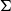, what is the maximum number of copies of H in an n-vertex graph G that embeds in? We show that the answer is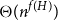, where f(H) is a graph invariant called the ‘flap-number’ of H, which is independent of. This simultaneously answers two open problems posed by Eppstein ((1993) J. Graph Theory17(3) 409–416.). The same proof also answers the question for minor-closed classes. That is, if H is a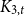minor-free graph, then the maximum number of copies of H in an n-vertexminor-free graph G is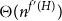, where f′(H) is a graph invariant closely related to the flap-number of H. Finally, when H is a complete graph we give more precise answers.

]]>
https://dx.doi.org/10.1017/S0963548321000560?rft_dat=source%3Ddrssdoi:10.1017/S0963548321000560Subgraph densities in a surface81283910.1017/S0963548321000560https://dx.doi.org/10.1017/S0963548321000560?rft_dat=source%3Ddrss2022-01-11Combinatorics, Probability and Computing2022-01-11Huynh, TonyJoret, GwenaëlWood, David R.
<![CDATA[Unusually large components in near-critical Erdős–Rényi graphs via ballot theorems]]>

We consider the near-critical Erdős–Rényi random graph G(n, p) and provide a new probabilistic proof of the fact that, when p is of the form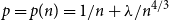and A is large,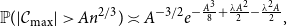where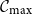is the largest connected component of the graph. Our result allows A and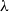to depend on n. While this result is already known, our proof relies only on conceptual and adaptable tools such as ballot theorems, whereas the existing proof relies on a combinatorial formula specific to Erdős–Rényi graphs, together with analytic estimates.

]]>
https://dx.doi.org/10.1017/S0963548321000584?rft_dat=source%3Ddrssdoi:10.1017/S0963548321000584Unusually large components in near-critical Erdős–Rényi graphs via ballot theorems84086910.1017/S0963548321000584https://dx.doi.org/10.1017/S0963548321000584?rft_dat=source%3Ddrss2022-02-11Combinatorics, Probability and Computing2022-02-11De Ambroggio, UmbertoRoberts, Matthew I.
<![CDATA[Short proofs for long induced paths]]>

We present a modification of the Depth first search algorithm, suited for finding long induced paths. We use it to give simple proofs of the following results. We show that the induced size-Ramsey number of paths satisfies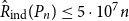, thus giving an explicit constant in the linear bound, improving the previous bound with a large constant from a regularity lemma argument by Haxell, Kohayakawa and Łuczak. We also provide a bound for the k-colour version, showing that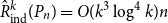. Finally, we present a new short proof of the fact that the binomial random graph in the supercritical regime,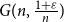, contains typically an induced path of length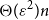.

]]>
https://dx.doi.org/10.1017/S0963548322000013?rft_dat=source%3Ddrssdoi:10.1017/S0963548322000013Short proofs for long induced paths87087810.1017/S0963548322000013https://dx.doi.org/10.1017/S0963548322000013?rft_dat=source%3Ddrss2022-02-11Combinatorics, Probability and Computing2022-02-11Draganić, NemanjaGlock, StefanKrivelevich, Michael
<![CDATA[Refined universality for critical KCM: lower bounds]]>

We study a general class of interacting particle systems called kinetically constrained models (KCM) in two dimensions tightly linked to the monotone cellular automata called bootstrap percolation. There are three classes of such models, the most studied being the critical one. In a recent series of works by Martinelli, Morris, Toninelli and the authors, it was shown that the KCM counterparts of critical bootstrap percolation models with the same properties split into two classes with different behaviour. Together with the companion paper by the first author, our work determines the logarithm of the infection time up to a constant factor for all critical KCM, which were previously known only up to logarithmic corrections. This improves all previous results except for the Duarte-KCM, for which we give a new proof of the best result known. We establish that on this level of precision critical KCM have to be classified into seven categories instead of the two in bootstrap percolation. In the present work, we establish lower bounds for critical KCM in a unified way, also recovering the universality result of Toninelli and the authors and the Duarte model result of Martinelli, Toninelli and the second author.

]]>
https://dx.doi.org/10.1017/S0963548322000025?rft_dat=source%3Ddrssdoi:10.1017/S0963548322000025Refined universality for critical KCM: lower bounds87990610.1017/S0963548322000025https://dx.doi.org/10.1017/S0963548322000025?rft_dat=source%3Ddrss2022-03-03Combinatorics, Probability and Computing2022-03-03Hartarsky, IvailoMarêché, Laure
<![CDATA[On tripartite common graphs]]>

A graph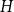is common if the number of monochromatic copies ofin a 2-edge-colouring of the complete graph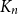is asymptotically minimised by the random colouring. Burr and Rosta, extending a famous conjecture of Erdős, conjectured that every graph is common. The conjectures of Erdős and of Burr and Rosta were disproved by Thomason and by Sidorenko, respectively, in the late 1980s. Collecting new examples of common graphs had not seen much progress since then, although very recently a few more graphs were verified to be common by the flag algebra method or the recent progress on Sidorenko’s conjecture. Our contribution here is to provide several new classes of tripartite common graphs. The first example is the class of so-called triangle trees, which generalises two theorems by Sidorenko and answers a question of Jagger, Šťovíček, and Thomason from 1996. We also prove that, somewhat surprisingly, given any tree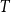, there exists a triangle tree such that the graph obtained by addingas a pendant tree is still common. Furthermore, we show that adding arbitrarily many apex vertices to any connected bipartite graph on at most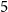vertices yields a common graph.

]]>
https://dx.doi.org/10.1017/S0963548322000074?rft_dat=source%3Ddrssdoi:10.1017/S0963548322000074On tripartite common graphs90792310.1017/S0963548322000074https://dx.doi.org/10.1017/S0963548322000074?rft_dat=source%3Ddrss2022-05-25Combinatorics, Probability and Computing2022-05-25Grzesik, AndrzejLee, JoonkyungLidický, BernardVolec, Jan